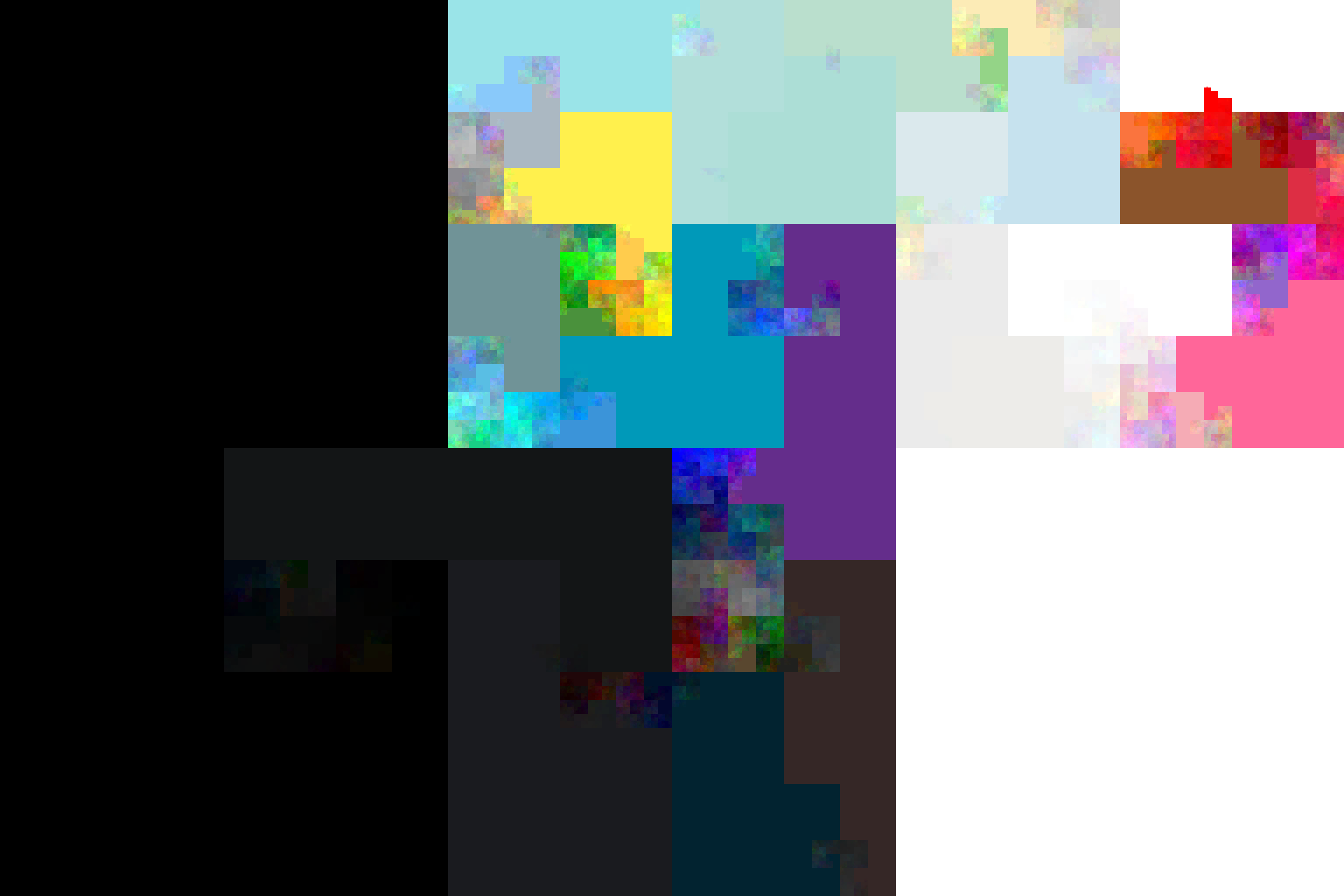I collected tweets of $$>8'000'000$$ Twitter users for an academic project. But Twitter does not only give you the tweets, but also many more data like the profile background color of users. It would be a shame to let these data go to waste, so I decided to process them into digital art. I wanted to show all the colors in one picture and group similar colors close to each other. This turned out to be much less trivial than I expected, since the space in which the colors live is the three dimensional RGB cube, but my image is only two dimensional. There is no “correct” way to project the colors down.

Here, I decided to put a 2D Hilbert curve through the image and paint the colors in the order they are encountered by a 3D Hilbert curve in the RGB cube. Ignoring the two default colors #F5F8FA and #C0DEED, this produces this image:And thanks to the Python packages hilbertcurve and pypng the code needed to generate this image is quite harmless:

from math import ceil, sqrt, log2

from hilbertcurve.hilbertcurve import HilbertCurve
import png

"""
turn an RGB string like #C0DEED into a tuple of integers,
i.e., coordinates of the RGB cube
"""
def str2rgb(s):
s = s.strip("#")
return (int(s[0:2], 16), int(s[2:4], 16), int(s[4:6], 16))

"""
color_histogram is a dict mapping an rgb string like #F5F8FA
to the number of usages of this color
"""
def plot_background_colors(color_histogram, filename="colors.png"):
defaults = {"F5F8FA", "C0DEED"}

data = {str2rgb(rgb): d for rgb, d in color_histogram if rgb not in defaults}

# calculate the size of the resulting image
# for a 2D Hilbert curve, it mus be square with a width, which is a power of 2
num_pixels = sum(data.values())
min_width = ceil(sqrt(num_pixels))
exponent = ceil(log2(min_width))
width = 2**exponent

# output buffer for a width x width png, with 4 color values per pixel
buf = [[0 for _ in range(4 * width)] for _ in range(width)]

hc2 = HilbertCurve(exponent, 2)
# there are 256 = 2^8 values in each direction of the RGB cube
hc3 = HilbertCurve(8, 3)

sorted_rgbs = sorted(data.keys(), key=lambda x: hc3.distance_from_point(x))

idx = 0
for rgb in sorted_rgbs:
for _ in range(data[rgb]):
# get the coordinate of the next pixel
x, y = hc2.point_from_distance(idx)
# assign the RGBA values to the pixel
buf[x][4 * y] = rgb
buf[x][4 * y + 1] = rgb
buf[x][4 * y + 2] = rgb
buf[x][4 * y + 3] = 255

idx += 1

png.from_array(buf, 'RGBA').save(filename)


The input histogram was in my case just a simple SQL query away:

SELECT profile_background_color, COUNT(profile_background_color) FROM users
GROUP BY profile_background_color;